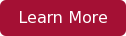<iframe src="https://5923915.fls.doubleclick.net/activityi;src=5923915;type=hbx_core;cat=hbx_b0;dc_lat=;dc_rdid=;tag_for_child_directed_treatment=;ord=1;num=1?" width="1" height="1" frameborder="0" style="display:none">In finance, we say that the value of something today is the present value of its discounted cash flows. We use this to value everything, and finance courses (like ours) give examples of valuing projects and businesses.

## What is a Bond?

A bond is a debt investment in which an investor loans money to an entity (typically corporate or governmental) which borrows the funds for a defined period of time at a variable or fixed interest rate. Bonds are used by companies, municipalities, states and sovereign governments to raise money and finance a variety of projects and activities. Owners of bonds are debtholders, or creditors, of the issuer.

Source: Investopedia.com

Turns out, bonds can be valued exactly the same way. Bonds, however, can be a bit obscured by finance jargon, so we'll need to break through a bit to figure out their value.

Let's take an imaginary bond: it has a face value of \$1000, an annual coupon of 3%, and a maturity date in 30 years. What does all that mean?

What is means is that the company or country that owes the bond will pay the bond holder 3% of the face value of \$1000 (\$30) every year for 30 years, at which point they will pay the bond holder the full \$1000 face value.

That gives us our cash flows. We have a series of 30 cash flows, one each year of \$30, and then we have one cash flow, 30 years from now, of \$1000.

Now we need to use our discounting formula:

Cash Flow ÷ (1+r)t

We have the cash flows and we have the number of years for each of them (called "t" in that equation). We need the "r", which is the interest rate. Which should we use? What we do is we use the current interest rate for similar 30-year bonds today. Since we're making up these numbers for the purpose of this example, it doesn't really matter what we use - we can say it's 5%.

So now we can value the various cash flows. First, we have the coupon payments:

30 ÷ (1+.05)1 + 30 ÷ (1+.05)2... + 30 ÷ (1+.05)30

And then we have the final face value payment, in thirty years:

1000 ÷ (1+.05)30

Together, these total the price - \$692.55. This price will ensure that the bond holder receives an annual return of 5% over the life of the bond.

Now that we have our price, we can play with some of our assumptions to see how things change. What if the prevailing market interest rate were 4% instead of 5%? In that case, the price of the bond would be \$827.08. If it were 6% instead of 5%, the price would be \$587.06. So, one thing to remember is that the price of a bond is inversely related to the interest rate - when interest rates go up, the price of bonds goes down, and vice versa. When the price of the bond is beneath the face value, we say that the bond is "trading at a discount." When the price of the bond is above the face value, we say the bond is "trading at a premium."

This can be important if you don't want to actually own the bond for 30 years. If you want to hold the bond for 5 years, then you'd receive \$30 per year for 5 years, and then you'd receive that price of the bond at that time, which will depend on the current interest rates at the time. This is why, while some long term bonds (like government Treasury bonds) can be considered "risk free" over their full lifetime, they will often vary a great deal in value on a year-to-year basis.

Want to better understand finance? Interested in developing a toolkit to make smarter financial decisions? Learn more about the HBX course, Leading with Finance.